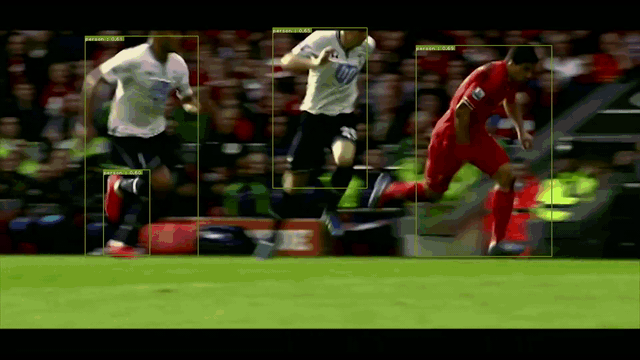# signatrix / efficientdet

(Pretrained weights provided) EfficientDet: Scalable and Efficient Object Detection implementation by Signatrix GmbH

https://signatrix.com/

Geek Repo

Github PK Tool

# EfficientDet: Scalable and Efficient Object Detection

## Introduction

Here is our pytorch implementation of the model described in the paper EfficientDet: Scalable and Efficient Object Detection paper (Note: We also provide pre-trained weights, which you could see at ./trained_models)An example of our model's output.

## Datasets

Dataset Classes #Train images #Validation images
COCO2017 80 118k 5k

Create a data folder under the repository,

``````cd {repo_root}
mkdir data
``````
• COCO: Download the coco images and annotations from coco website. Make sure to put the files as the following structure:
``````COCO
├── annotations
│   ├── instances_train2017.json
│   └── instances_val2017.json
│── images
├── train2017
└── val2017
``````

## How to use our code

With our code, you can:

• Train your model by running python train.py
• Evaluate mAP for COCO dataset by running python mAP_evaluation.py
• Test your model for COCO dataset by running python test_dataset.py --pretrained_model path/to/trained_model
• Test your model for video by running python test_video.py --pretrained_model path/to/trained_model --input path/to/input/file --output path/to/output/file

## Experiments

We trained our model by using 3 NVIDIA GTX 1080Ti. Below is mAP (mean average precision) for COCO val2017 dataset

Average Precision IoU=0.50:0.95 area= all maxDets=100 0.314
Average Precision IoU=0.50 area= all maxDets=100 0.461
Average Precision IoU=0.75 area= all maxDets=100 0.343
Average Precision IoU=0.50:0.95 area= small maxDets=100 0.093
Average Precision IoU=0.50:0.95 area= medium maxDets=100 0.358
Average Precision IoU=0.50:0.95 area= large maxDets=100 0.517
Average Recall IoU=0.50:0.95 area= all maxDets=1 0.268
Average Recall IoU=0.50:0.95 area= all maxDets=10 0.382
Average Recall IoU=0.50:0.95 area= all maxDets=100 0.403
Average Recall IoU=0.50:0.95 area= small maxDets=100 0.117
Average Recall IoU=0.50:0.95 area= medium maxDets=100 0.486
Average Recall IoU=0.50:0.95 area= large maxDets=100 0.625

## Results

Some predictions are shown below:

## Requirements

• python 3.6
• pytorch 1.2
• opencv (cv2)
• tensorboard
• tensorboardX (This library could be skipped if you do not use SummaryWriter)
• pycocotools
• efficientnet_pytorch